Checkout JEE MAINS 2022 Question Paper Analysis : Checkout JEE MAINS 2022 Question Paper Analysis :

# Thevenin's Theorem

Most commonly, we use Ohm’s law, Kirchhoff’s law to solve complex electrical circuits, but we must also be aware that there are many circuit analysis theorems using which we can calculate the current and voltage at any given point in a circuit. Among the various circuit theorems, Thevenin’s theorem is most commonly used. In this article, let us learn about Thevenin’s theorem in detail.

## Thevenin’s Theorem Explanation

Thevenin’s theorem states that it is possible to simplify any linear circuit, irrespective of how complex it is, to an equivalent circuit with a single voltage source and a series resistance.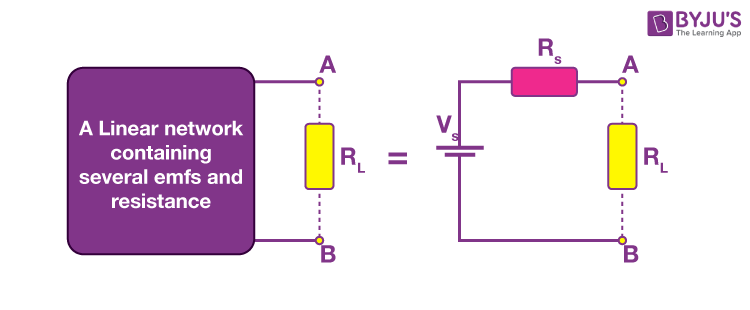A Thevenin equivalent circuit is shown in the image. In the image, we see that multiple resistive circuit elements are replaced by a single equivalent resistance Rs and multiple energy sources by an equivalent voltage source Vs.

### Thevenin’s Theorem Example

Let us understand Thevenin’s Theorem with the help of an example.

Example: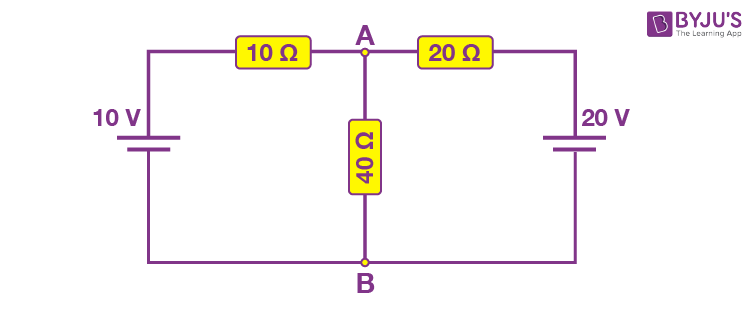Step 1: For the analysis of the above circuit using Thevenin’s theorem, firstly remove the load resistance at the centre, in this case, 40 Ω.

Step 2: Remove the voltage sources’ internal resistance by shorting all the voltage sources connected to the circuit, i.e. v = 0. If current sources are present in the circuit, then remove the internal resistance by open circuiting the sources. This step is done to have an ideal voltage source or an ideal current source for the analysis.

Step 3: Find the equivalent resistance. In the example, the equivalent resistance of the circuit is calculated as follows: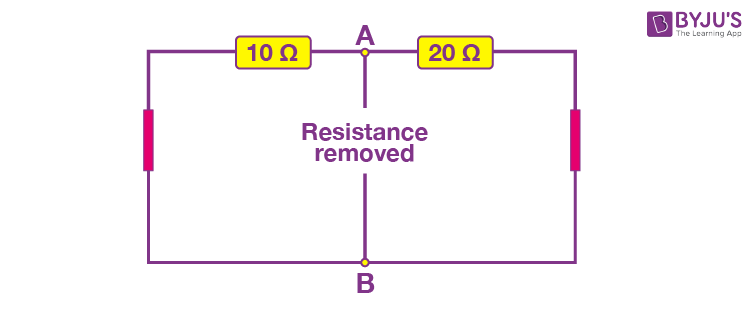With the load resistance removed and the voltage sources shorted, the equivalent resistance of the circuit is calculated as follows:

The resistor 10 Ω is parallel to 20 Ω, therefore the equivalent resistance of the circuit is:

$$\begin{array}{l}R_{T}=\frac{R_{1}\times R_{2}}{R_{1}+R_{2}}=\frac{20\times 10}{20+10}=6.67\,\Omega\end{array}$$

Step 4: Find the equivalent voltage.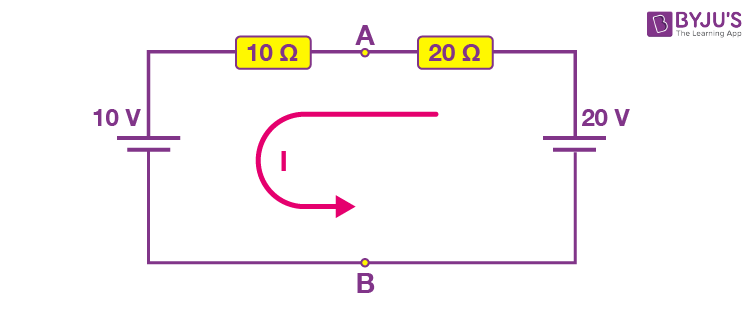To calculate the equivalent voltage, reconnect the voltage sources back into the circuit. Vs = VAB, therefore the current flowing around the loop is calculated as follows:

$$\begin{array}{l}I=\frac{V}{R}=\frac{20\,V-10\,V}{20\,\Omega+10\,\Omega}=0.33\,A\end{array}$$

The calculated current is common to both resistors, so the voltage drop across the resistors can be calculated as follows:

VAB = 20 – (20 Ω x 0.33 A) = 13.33 V

or,

VAB = 10 + (10 Ω x 0.33 A) = 13.33 V

The voltage drop across both resistors is the same.

Step 5: Draw the Thevenin’s equivalent circuit. The Thevenin’s equivalent circuit consists of a series resistance of 6.67 Ω and a voltage source of 13.33 V.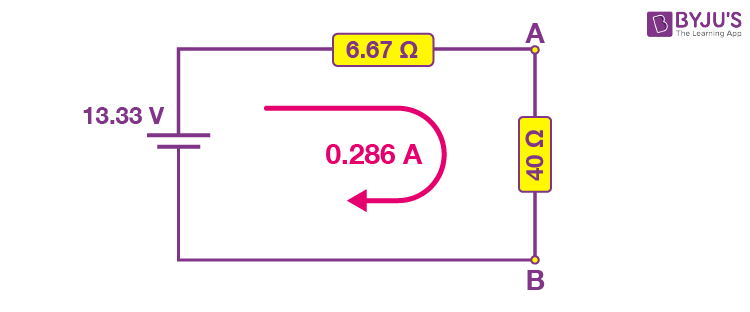The current flowing in the circuit is calculated using the formula below:

$$\begin{array}{l}I=\frac{V}{R}=\frac{13.33\,V}{6.67\,\Omega + 40\,\Omega}=0.286\,A\end{array}$$

Thevenin’s theorem can be applied to both AC and DC circuits. But it should be noted that this method can only be applied to AC circuits consisting of linear elements like resistors, inductors, capacitors. Like Thevenin’s equivalent resistance, Thevenin’s equivalent impedance is obtained by replacing all voltage sources with their internal impedances.

## Thevenin’s Theorem Solved Example

Find VTH, RTH and the load current IL flowing through and load voltage across the load resistor in the circuit below using Thevenin’s Theorem.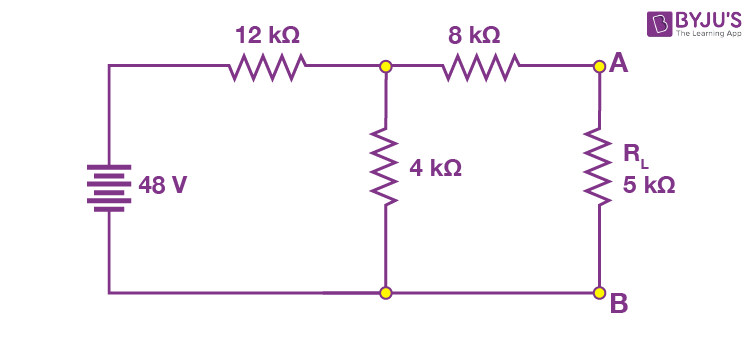Solution:

Step 1: Remove the 5 kΩ from the circuit.

Step 2: Measure the open-circuit voltage. This will give you the Thevenin’s voltage (VTH).

Step 3: We calculate Thevenin’s voltage by determining the current that flows through 12 kΩ and 4 kΩ resistors.

As both the resistors are in series, the current that flows across them can be calculated as follows:

I = 48 V /( 12 kΩ + 4 kΩ) = 3 mA

The voltage across the 4 kΩ resistors can be calculated as follows:

3 mA x 4 kΩ = 12 V

As there is no current flowing through the 8 kΩ resistor, so there is no voltage drop across it and hence the voltage across the terminals AB is same as the voltage across the 4 kΩ resistor. Therefore, 12 V will appear across the AB terminals. Hence, the Thevenin’s voltage, VTH = 12 V.

Step 4: Short the voltage sources as shown in the figure below: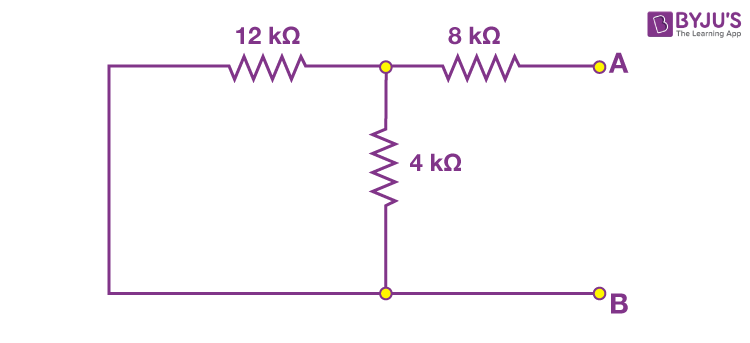Step 5: Calculate the Thevenin’s Resistance

By measuring the open circuit resistance, we can measure Thevenin’s resistance.

We notice that the 8 kΩ resistor is in series with the parallel connection of 12 kΩ and 4 kΩ resistors. Therefore, the equivalent resistance or the Thevenin’s resistance is calculated as follows:

8kΩ + (4k Ω || 12kΩ)

RTH = 8 kΩ + [(4 kΩ x 12 kΩ) / (4 kΩ + 12 kΩ)]

RTH = 8 kΩ + 3 kΩ

RTH = 11 kΩ

Step 6: Now, connect the RTH in series with Voltage Source VTH and the load resistor as shown in the figure.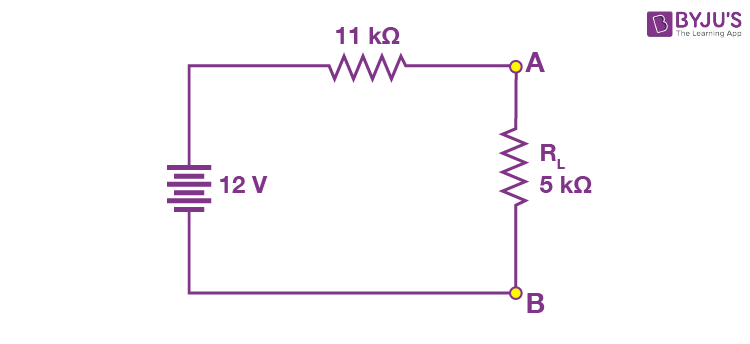Step 7: For the last step, calculate the load voltage and load current using Ohm’s law as follows:

IL = VTH / (RTH + RL)

IL = 12 V / (11 kΩ + 5 kΩ) = 12 V/16 kΩ = 0.75 mA

The load voltage is determined as follows:

VL = 0.75 mA x 5 kΩ = 3.75 V

## Thevenin Theorem Applications

• Thevenin’s theorem is used in the analysis of power systems.
• Thevenin’s theorem is used in source modelling and resistance measurement using the Wheatstone bridge.

## Thevenin Theorem Limitations

• Thevenin’s theorem is used only in the analysis of linear circuits.
• The power dissipation of the Thevenin equivalent is not identical to the power dissipation of the real system.

## Frequently Asked Questions – FAQs

### What is Thevenin’s Theorem?

Thevenin’s theorem states that it is possible to simplify any linear circuit, irrespective of how complex it is, to an equivalent circuit with a single voltage source and a series resistance.

### What is Thevenin Voltage?

The open-circuit voltage that appears across the two terminals is the Thevenin voltage.

### Where is Thevenin’s Theorem used?

Thevenin’s theorem is used in the analysis of power systems.

### Is Thevenin’s Theorem applicable to non-linear circuits?

No, Thevenin’s Theorem is not applicable to non-linear circuits.

### Is Thevenin’s Theorem applicable to AC circuits?

Yes, Thevenin’s theorem does not apply to AC circuits consisting of linear elements like resistors, inductors, and capacitors.

Stay tuned to BYJU’S and Fall in Love with Learning!

Test your Knowledge on Thevenin's Theorem

#### 1 Comment

1. Pankaj verma

Good